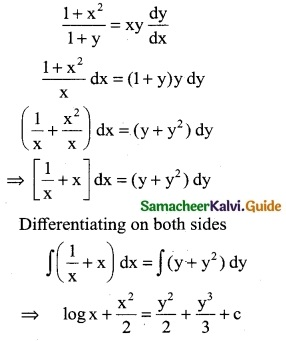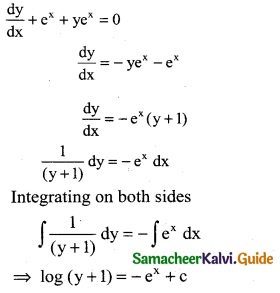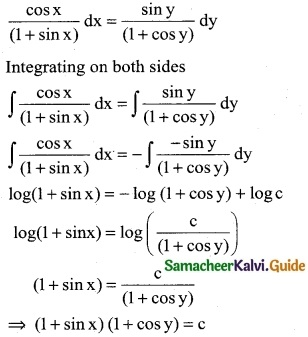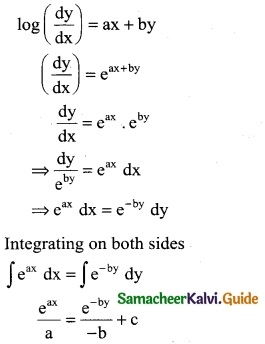Tamilnadu State Board New Syllabus Samacheer Kalvi 12th Business Maths Guide Pdf Chapter 4 Differential Equations Ex 4.2 Text Book Back Questions and Answers, Notes.

## Tamilnadu Samacheer Kalvi 12th Business Maths Solutions Chapter 4 Differential Equations Ex 4.2

Question 1.
Solve:
(i) $$\frac { dy }{dx}$$ = aey
Solution:
$$\frac { dy }{dx}$$ = aey
$$\frac { dy }{e^y}$$ = adx ⇒ e-y dy = adx
Integrating on both sides
$$\frac { e^y }{(-1)}$$ = ax + c
-e-y = ax + c ⇒ e-y + ax + c = 0(ii) $$\frac { 1+x^2 }{1+y}$$ = xy $$\frac { dy }{dx}$$
Solution:Question 2.
y(1 – x) – x $$\frac { dy }{dx}$$ = 0
Solution:Question 3.
(i) ydx – xdy = 0 dy
Solution:
ydx – xdy = 0
ydx = xdy
$$\frac { 1 }{x}$$ dx = $$\frac { 1 }{y}$$ dy
Integrating on both sides
∫$$\frac { 1 }{x}$$dx = ∫$$\frac { 1 }{y}$$dy
log x = log y + log c
log x = log cy
⇒ x = cy

(ii) $$\frac { dy }{dx}$$ + ex + yex = 0
Solution:Question 4.
Solve : cosx (1 + cosy) dx – siny (1 + sinx) dy = 0
Solution:
cos x (1 + cos y) dx – sin y (1 + sin x) dy = 0
cos x (1 + cos y) dx = sin y (1 + sin x) dyQuestion 5.
Solve: (1 – x) dy – (1 + y) dx = 0
Solution:
(1 – x) dy – (1 + y) dx = 0
(1 – x) dy = (1 + y) dx
$$\frac { dy }{(1+y)}$$ = $$\frac { dx }{(1-x)}$$
Integrating on both sidesQuestion 6.
Solve:
(i) $$\frac { dy }{dx}$$ = y sin 2x
Solution:(ii) log($$\frac { dy }{dx}$$) = ax + by
Solution:Question 7.
Find the curve whose gradient at any point P (x, y) on it is $$\frac { x-a }{y-b}$$ and which passes through the origin.
Solution:
The gradient of the curve at P (x, y)
$$\frac { dy }{dx}$$ = $$\frac { x-a }{y-b}$$
(y – b) dy = (x – a) dx
Integrating on both sides
∫(y – b)dy = ∫(x – a)dx
⇒ $$\frac { (y-b)^2 }{2}$$ = $$\frac { (x-a)^2 }{2}$$ + c
(Multiply each term by 2)
∴ (y – b)² = (x – a)² + 2c ……… (1)
Since the curve passes through the origin (0, 0)
eqn (1) (0 – b)² = (0 – a)² + 2c
b² = a² + 2c
b² – a² = 2c ………. (2)
Substitute eqn (2) in eqn (1)
(y – b)² = (x – a)² + b² – a²